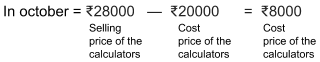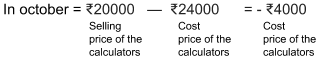# Profit and Loss

Go back to  'Commercial Math'

Money is one of the most important engines in the professional world. At the end of the day, most things that you do to interact with the outside world has a monetary value attached to it. Which is why it is crucial that we are well versed with handling all the calculations that revolve around money.  Profit and Loss are the basic driving forces of the market, and here you will understand how to wield the power of mathematics and apply it to the commercial world.

Let’s take the simple example of buying and selling a vehicle. Suppose you bought a furniture for ₹50,000. You hardly used the furniture and sold it all for ₹45,000.  In this case you have made a loss of ₹5000. Had you sold it for ₹55,000 you would have made a profit of ₹5000.

Here, the initial amount that you paid for the scooter, ₹50,000 is the $$\text{Cost Price (C.P.).}$$ The price that you sold it for is called the $$\text{Selling Price (S.P.).}$$

Let’s try and understand this better using an example,

### Case 1

In October, a shopkeeper bought 40 scientific calculators (in bulk) for ₹20,000. He then sold each calculator for ₹700.

• Cost price of the calculators = ₹20,000
• Selling  price of the calculators = ₹28,000When the selling price is greater than the cost price, then $$\text{SP} - \text{CP}$$ will be positive, then we say that a profit is made.

### Case 2

In November, the shopkeeper bought 40 more scientific calculators for ₹600 each. He then sold all of them at ₹20,000.

• Cost price of the calculators = ₹24,000
• Selling  price of the calculators = ₹20,000When the selling price is less than the cost price, then SP – CP will be negative, then we say that a loss is made

## Profit and loss percentage

Percentage is something that is applicable to most of the concepts. They are an easy way to gauge an increase or decrease in an amount. Profits and losses are no exceptions. As a general rule of thumb, the higher the profit percentage, the better the lower the loss percentage the better. Let’s try and understand this with the help of an example.

### Formula

\begin{align}{Profit \over Cost \; Price} \times 100 = Profit \; Percentage \end{align}

\begin{align}{Loss \over Cost \; Price} \times 100 = Loss \; Percentage \end{align}

Example 1

Mia bought a game for ₹750 and sold it for ₹875. Find Mia's profit percentage.

\begin{align}\text{Profit} &= \text{Selling Price} - \text{Cost Price} \\ \text{Profit} &= ₹875 - ₹750 \\ \text{Profit} &= ₹125 \end{align}

\begin{align} \text{Profit Percentage} &= {\text{Profit} \over \text{Cost Price}} \times 100 \\ &= {125 \over 750} \times 100 \\ &= {16.6\%} \end{align}

Example 2

Rohan purchased a table for ₹4500 and due to some scratches on its top he had to sell it for ₹4000. Find his loss per cent.

\begin{align}\text{Loss} &= \text{Selling Price} - \text{Cost Price} \\ \text{Loss} &= ₹4000 - ₹4500 \\ \text{Loss} &= -₹500 \end{align}

\begin{align} \text{Loss Percentage} &= {\text{Loss} \over \text{Cost Price}} \times 100 \\ &= {-500 \over 4500} \times 100 \\ &= {-11.1 \%} \end{align}

## Tips and Tricks

• It is often the case that we buy something and spend extra money on repairing it, transporting it, etc. These extra expenses are called overhead expenses. Overhead expenses add to the cost price. The repairs/upgrades etc., add to the cost price of an item.
• Often when calculating the profit or loss percentage, students take the selling price in the denominator instead of the cost price. Reiterate often that profit and loss percentages are always calculated over cost price (CP)
$$\text{Profit } \% = (\text{Profit } \div \text{Cost Price}) \times 100$$